# 06-240/Classnotes For Thursday, September 21

## Force Vectors

A force has a direction and a magnitude.

1. There is a special force vector called 0.
3. They can be multiplied by any scalar.

#### Properties

(convention: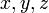$x,y,z$ are vectors;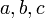$a,b,c$ are scalars)

1.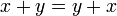$x+y=y+x$
2.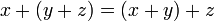$x+(y+z)=(x+y)+z \$
3.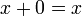$x+0=x \$
4.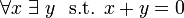$\forall x\; \exists\ y \ \mbox{ s.t. }x+y=0$
5.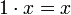$1\cdot x=x \$
6.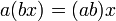$a(bx)=(ab)x \$
7.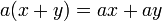$a(x+y)=ax+ay \$
8.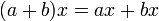$(a+b)x=ax+bx \$
##### Definition

Let F be a field "of scalars". A vector space over F is a set V, of "vectors", along with two operations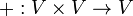$+: V \times V \to V$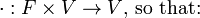$\cdot: F \times V \to V \mbox{, so that:}$
1.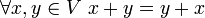$\forall x,y \in V\ x+y=y+x$
2.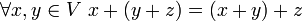$\forall x,y \in V\ x+(y+z)=(x+y)+z$
3.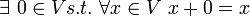$\exists\ 0 \in V s.t.\; \forall x \in V\ x+0=x$
4.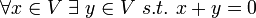$\forall x \in V\; \exists\ y \in V\ s.t. \ x+y=0$
5.$1\cdot x=x\$
6.$a(bx)=(ab)x\$
7.$a(x+y)=ax+ay\$
8.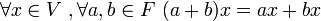$\forall x \in V\ ,\forall a,b \in F\ (a+b)x=ax+bx$

9.$x \mapsto \vert x\vert \in \mathbb{R} \ \vert x+y\vert \le \vert x\vert+\vert y\vert$

#### Examples

Ex.1.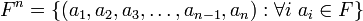$F^n= \lbrace(a_1,a_2,a_3,\ldots,a_{n-1},a_n):\forall i\ a_i \in F \rbrace$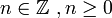$n \in \mathbb{Z}\ , n \ge 0$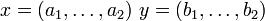$x=(a_1,\ldots,a_2)\ y=(b_1,\ldots, b_2)\$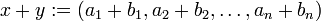$x+y:=(a_1+b_1,a_2+b_2,\ldots,a_n+b_n)\$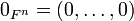$0_{F^n}=(0,\ldots,0)$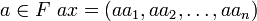$a\in F\ ax=(aa_1,aa_2,\ldots,aa_n)$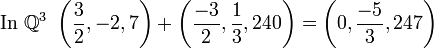$\mbox{In } \mathbb{Q}^3 \ \left( \frac{3}{2},-2,7\right)+\left( \frac{-3}{2}, \frac{1}{3},240\right)=\left(0, \frac{-5}{3},247\right)$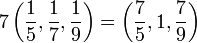$7\left( \frac{1}{5},\frac{1}{7},\frac{1}{9}\right)=\left( \frac{7}{5},1,\frac{7}{9}\right)$
Ex.2.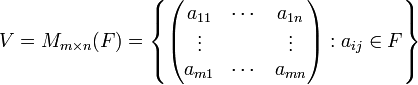$V=M_{m\times n}(F)=\left\lbrace\begin{pmatrix} a_{11} & \cdots & a_{1n} \\ \vdots & & \vdots \\ a_{m1} & \cdots & a_{mn}\end{pmatrix}: a_{ij} \in F \right\rbrace$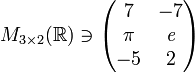$M_{3\times 2}( \mathbb{R})\ni \begin{pmatrix} 7 & -7 \\ \pi & \mathit{e} \\ -5 & 2 \end{pmatrix}$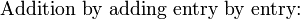$\mbox{Addition by adding entry by entry:}$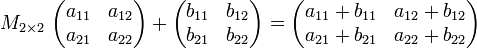$M_{2\times 2}\ \begin{pmatrix} a_{11} & a_{12} \\ a_{21} & a_{22} \end{pmatrix}+\begin{pmatrix} b_{11} & b_{12} \\ b_{21} & b_{22} \end{pmatrix}=\begin{pmatrix} {a_{11}+b_{11}} & {a_{12}+b_{12}} \\ {a_{21}+b_{21}} & {a_{22}+b_{22}} \end{pmatrix}$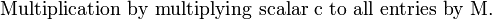$\mbox{Multiplication by multiplying scalar c to all entries by M.}$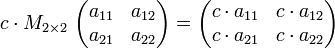$c\cdot M_{2\times 2}\ \begin{pmatrix} a_{11} & a_{12} \\ a_{21} & a_{22} \end{pmatrix}=\begin{pmatrix} c\cdot a_{11} & c\cdot a_{12} \\ c\cdot a_{21} & c\cdot a_{22} \end{pmatrix}$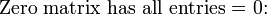$\mbox{Zero matrix has all entries = 0:}$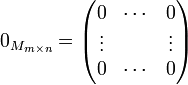$0_{M_{m\times n}}=\begin{pmatrix} 0 & \cdots & 0 \\ \vdots & & \vdots \\ 0 & \cdots & 0\end{pmatrix}$
Ex.3.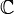$\mathbb{C}$ form a vector space over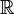$\mathbb{R}$.
Ex.4.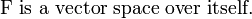$\mbox{F is a vector space over itself.}$
Ex.5.$\mathbb{R}$ is a vector space over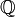$\mathbb{Q}$.
Ex.6.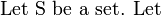$\mbox{Let S be a set. Let}$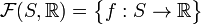$\mathcal{F}(S,\mathbb{R})=\big\{f:S\to \mathbb{R} \big\}$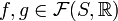$f,g \in \mathcal{F}(S,\mathbb{R})$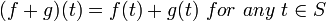$(f+g)(t)=f(t)+g(t)\ for\ any\ t\in S$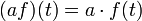$(af)(t)=a\cdot f(t)\$